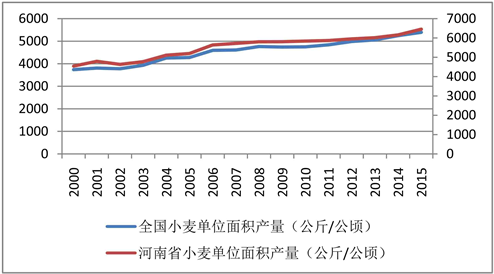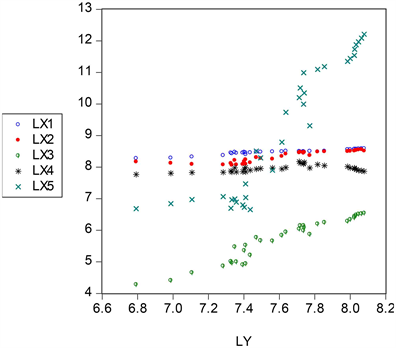﻿ 河南省小麦产量影响因素研究

# 河南省小麦产量影响因素研究 Study on the Factors Affecting Wheat Yield in Henan Province

Abstract: Henan is an important grain producing area in the country and an important production area for wheat in the country. The stability of wheat production in Henan Province is not only important for the province’s grain production, but also important for safeguarding national food supply and maintaining national food security. This paper uses multiple linear regression based on data on production factors such as wheat yield, wheat planting area, effective irrigated area, fertilizer use, number of agricultural machinery, agricultural employment, agricultural technology advancement, and agricultural policy support in Henan Province since 1980-2013. Analysis and stepwise regression analysis were used to analyze and predict wheat production factors and yields in Henan Province.

1. 河南省农业生产现状及问题Figure 1. Yield of wheat per unit area in China and Henan Province

1.1. 耕地面积受限

1.2. 农业环境污染严重

1.3. 农业政策不完善

2. 多元线性模型分析

2.1. 模型的设定Figure 2. Variable scatter plot

$\mathrm{ln}{Y}_{i}=C+\mathrm{ln}{X}_{1i}+\mathrm{ln}{X}_{2i}+{\beta }_{3}\mathrm{ln}{X}_{3i}+\mathrm{ln}{X}_{4i}+{\beta }_{5}\mathrm{ln}{X}_{5i}+{X}_{6i}+{\mu }_{i}$

2.2. 实证过程

2.2.1. OLS回归Table 1. Preliminary regression results

2.2.2. 异方差检验Table 2. Results of heteroscedasticity test

2.2.3. 相关性检验Table 3. Variable correlation test resultsTable 4. Univariate stepwise regression resultsTable 5. Multivariate stepwise regression resultsTable 6. Major variable regression results

$\begin{array}{l}\mathrm{ln}{\stackrel{^}{Y}}_{i}=-4.448639+1.193948\mathrm{ln}{X}_{1}+0.331977\mathrm{ln}{X}_{3}+0.102591{X}_{6}\\ \text{ }\text{ }\text{ }\left(0.1418\right)\text{ }\text{ }\left(0.0033\right)\text{ }\text{ }\text{ }\text{\hspace{0.17em}}\text{\hspace{0.17em}}\left(0.0000\right)\text{ }\text{ }\text{\hspace{0.17em}}\text{\hspace{0.17em}}\text{\hspace{0.17em}}\text{\hspace{0.17em}}\left(0.0001\right)\\ {R}^{2}=0.967869,{\stackrel{¯}{R}}^{2}=0.964655,F=301.2213,DW=1.86509\end{array}$

3. 实证结果分析及政策建议

3.1. 实证结果分析

3.2. 提高河南省小麦产量的对策建议

3.2.1. 保证耕地面积

3.2.2. 科学合理施用化肥

3.2.3. 加大政策对农业生产的支持力度

 卢峰. 2017年河南小麦市场情况分析及2018年展望[J]. 现代面粉工业, 2018, 32(2): 40-43.

 储志英. 小麦生产中存在的问题及对策[J]. 现代农业科技, 2017(21): 56-57.

 宋九林. 小麦生产过程中存在的问题与对策[J]. 种业导刊, 2017(6): 25-26.

 靳冰, 杨志豪, 杨朝民, 袁志平. 河南省小麦生产发展的新形势及存在的问题[J]. 南方农业, 2018, 12(8): 92-93.

 高洪泽, 赵平, 秦海英, 赵继文. 濮阳市小麦生产现状及技术需求[J]. 农业科技通讯, 2016(6): 8-10.

 高鸣, 宋洪远. 补贴减少了粮食生产效率损失吗?——基于动态资产贫困理论的分析[J]. 管理世界, 2017(9): 85-100.

 张伟, 景丽, 上官彩霞, 孙建军. 河南小麦供给侧结构性改革的思考与对策[J]. 农业经济, 2017(12): 3-5.

Top